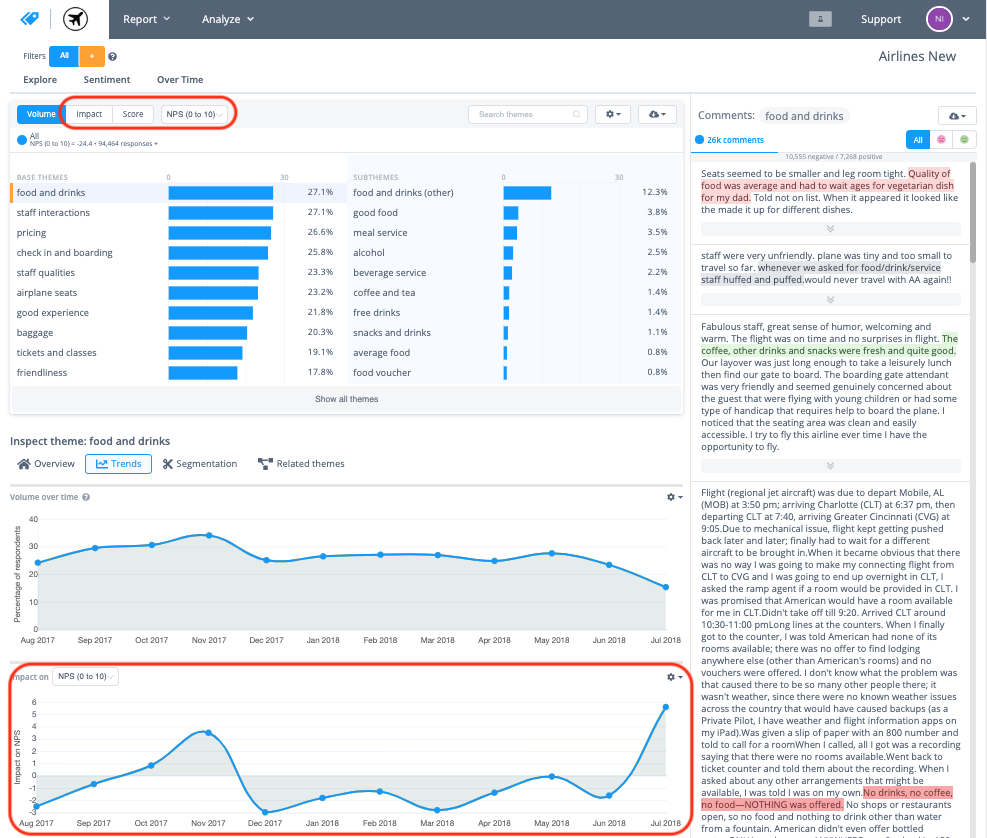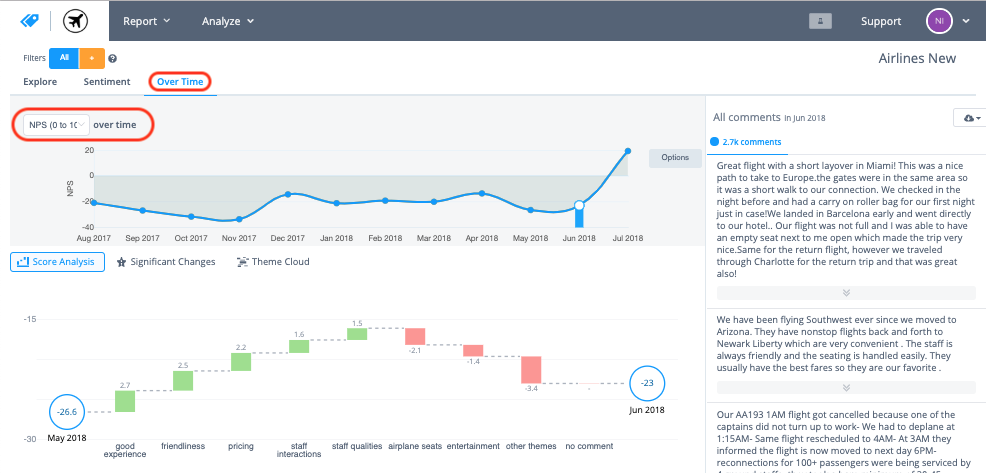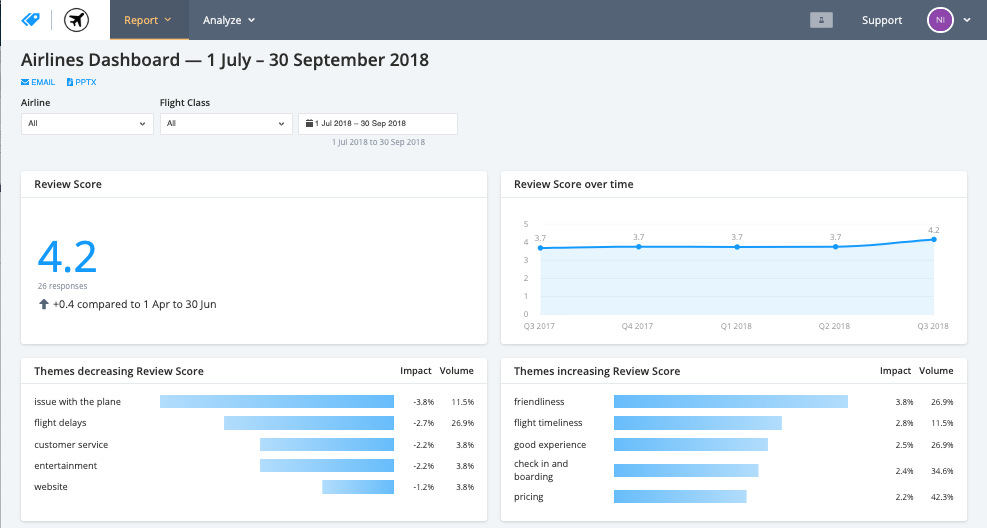# Example scores or metrics

Thematic can associate the themes found in your data with a variety of scores or metrics like NPS or CSAT. Thematic can then tell you what themes are driving this score positively or negatively. Here are some example scores Thematic can use in your data.

#### Sentiment

Thematic can analyze the language and terminology used in each response to determine if a response is positive and happy, or negative and angry. Each response is given a score between -1 and +1. -1 is the most negative, 0 is neutral and +1 is positive. Using this Thematic can calculate, what's the average sentiment of your dataset, what themes are affecting the average sentiment the most, and what themes cause the biggest negative and positive sentiment from responses.

Example: The average sentiment of your data set is -0.1, and the theme "Cancellations" has the most negative average sentiment.

#### Net Promoter Score (NPS)

Thematic can use a Net Promoter Score (0-10 How likely are you to recommend a product or service to your friends and family) to calculate what the total NPS of the dataset is, and how themes are impacting that NPS and by how much.

Example: Your NPS is 60, and because of responses mentioning "Cancellations" that NPS is 5 points lower.

#### Customer Satisfaction (CSAT)

Thematic can use a Customer Satisfaction Score (0-5 or 0-10) left by each response to calculate what the average CSAT is, and how themes are impacting that CSAT and by how much.

Example: Your average CSAT is 7 out of 10, and because of responses mentioning "Cancellations" that CSAT is 0.5 points lower.

#### Score Thresholds

Thematic can calculate the number of responses that fall into certain criteria and use that as a score. eg. what percentage of responses left you an 8 or greater out of 10=. Thematic can then tell you what themes are impacting that percentage and by how much.

Example: 80% of responses leave a 8 or greater out of 10, and because of responses mentioning "Cancellations" that percentage is 10% lower.

#### First Call Resolution (FCR)

Thematic can use a First Call Resolution note with each response (Yes my issue was resolved with one call, or No my issue wasn't resolved with one call) to calculate what the percentage of responses have their issue resolved on the first call/contact point, and how themes are impacting that percentage and by how much. THis is similar to Score thresholds but don't need a numeric score initially.

Example: Your average FCR is 75%, and because of responses mentioning "Cancellations" that FCR is 5% lower.

#### Response or Resolution time

Thematic can use response or resolution time included with each response (Number of hours or days) to calculate what the average response or resolution time is, and how themes are impacting that time and by how much.

Example: The average resolution time is 20 hours, and because of responses mentioning "Cancellations" the average time is 1 hour slower.

#### Monetary Value

Thematic can use a monetary value included with each response (Number of dollars spent on tickets or products) to calculate what the average monetary value of each response is, and how themes are impacting that amount and by how much.

Example: The average response spend was \$80, and because of responses mentioning "Cancellations" the average spend is \$5 less.

# In the tools

Your metrics can be selected and viewed in the Explore tool next to theme volume, and in themes trends. It can also be selected in the overtime tool. Metrics can also be displayed in your dashboards.